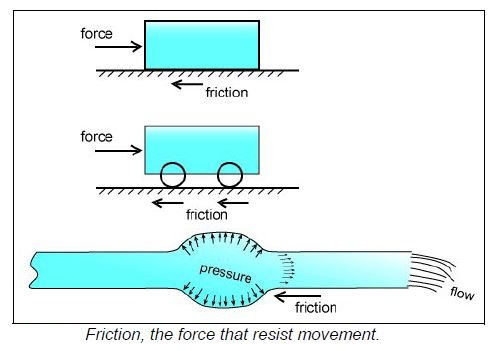# What is Friction in a Pump System ?

Friction is always present, even in fluids, it is the force that resists the movement of objects.When you move a solid on a hard surface, there is friction between the object and the surface. If you put wheels on it, there will be less friction. In the case of moving fluids such as water, there is even less friction but it can become significant for long pipes. Friction can be also be high for short pipes which have a high flow rate and small diameter as in the syringe example.

In fluids, friction occurs between fluid layers that are traveling at different velocities within the pipe (see below Figure ). There is a natural tendency for the fluid velocity to be higher in the center of the pipe than near the wall of the pipe. Friction will also be high for viscous fluids and fluids with suspended particles.Another cause of friction is the interaction of the fluid with the pipe wall, the rougher the pipe, the higher the friction.
Friction depends on:
• Average velocity of the fluid within the pipe
• Viscosity
• Pipe surface roughness
An increase in any one of these parameters will increase friction.

The amount of energy required to overcome the total friction loss within the system has to be supplied by the pump if you want to achieve the required flow rate. In industrial systems, friction is not normally a large part of a pump's energy output. For typical systems, it is around 25% of the total. If it becomes much higher then you should examine the system to see if the pipes are too small. However all pump systems are different, in some systems the friction energy may represent 100% of the pump's energy,

This is what makes pump systems interesting, there is a million and one applications for them. In household systems, friction can be a greater proportion of the pump energy output, maybe up to 50% of the total, this is because small pipes produce higher friction than larger pipes for the same average fluid velocity in the pipe (see the friction chart later in this tutorial).

Another cause of friction are the fittings (elbows, tees, y's, etc) required to get the fluid from point A to B. Each one has a particular effect on the fluid streamlines. For example in the case of the elbow (see below Figure ), the fluid streamlines that are closest to the tight inner radius of the elbow lift off from the pipe surface forming small vortexes that consume energy. This energy loss is small for one elbow but if you have several elbows and other fittings it can become significant. Generally speaking they rarely represent more than 30% of the total friction due to the overall pipe length.